MORE IN Mechanics of Materials
VTU Mechanical Engineering (Semester 3)
Mechanics of Materials
December 2013
Total marks: --
Total time: --
INSTRUCTIONS
(1) Assume appropriate data and state your reasons
(2) Marks are given to the right of every question
(3) Draw neat diagrams wherever necessary

1 (a) Define :
(i) True stress
(ii) Factor of safety
(iii) Poisson's ratio
(iv) Principle of super position
4 M
1 (b) A bar of uniform thickness 't' tapers uniformly from a width of b1 at one end to b2 at other end, in a length of 'L'. Find the expression for the change in length of the bar when subjected to an axial force P.
8 M
1 (c) A vertical circular steel bar of length 3l fixed at at both of its ends is loaded at intermediate sections by forces W and 2W as shown in fig. Q.2(c). Determine the end reactions if W=1.5kN.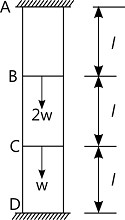8 M

2 (a) Define:
(i) Volumetric strain
(ii) Bulk modulus
2 M
2 (b) A bar of rectangular cross section shown in fig.Q.2(b)is subjected to stresses σx, σy and σz in x, y and z directions respectively. Show that if sum of these stresses is zero, there is no change in volume of the bar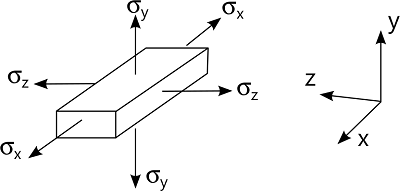9 M
2 (c) Rails are laid such that there is no stress in them at 24°C.If the rails are 32m long, determine
(i) The stress in the rails at 80°C, when there is no allowance for expansion
(ii) The stress in the rails at 80°C, when there is an expansion allowance of 8 mm per rail
(iii) The expansion allowance for no stress in the rail at 80°C
Coefficient of linear expansion α=11×10-6/°C and Young's modulus E=205GPa.
9 M

3 (a) Derive the expressions for normal and tangential stress on a plane inclined at 'θ' to the plane of stress in x-direction in a general two dimensional stress system and show that sum of normal stress in any two mutually perpendicular directions is constant.
12 M
3 (b) The state of stress in a two dimensionally stresses body is shown in fig.Q.3(b). Determine graphically (by drawing Mohr's circle), the principle stresses, principle planes, maximum shear stress and its planes.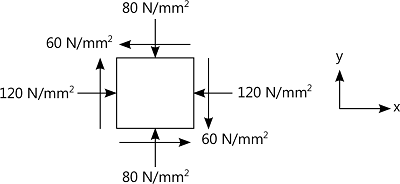8 M

4 (a) A beam of length l is simply supported at its ends. The beam carries a uniformly distributed load of w per unit run over the whole span. Find the strain energy stored by the beam.
6 M
4 (b) A water main 80cm diameter contains water at a pressure head of 100m. If the weight density of water is 9810N/m3, find the thickness of the metal required for the water main. Given the permissible stress as 20N/mm2.
6 M
4 (c) A pipe of 400mm internal diameter and 100mm thickness contains a fluid at a pressure of 8N/mm2. Find the maximum and minimum hoop stress across the section. Also, sketch the radial pressure distribution and hoop stress distributed across the section.
6 M

5 (a) Define a beam. Explain with simple sketches, different types of beams.
6 M
5 (b) Draw the shear force and bending moment diagrams for the overhanging beam carrying uniformly distributed load of 2kN/m over the entire length and a point load of 2 kN as shown in fig.Q5(b). Locate the point of contra flexure.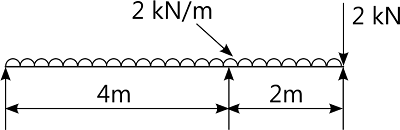14 M

6 (a) State the assumptions made in the theory of simple bending.
2 M
6 (b) A simply supported cast iron square beam of 800mm length and 15mm×15mm in section fails on applying a load of 360N at the mid span. Find the maximum uniformly distributed load that can be applied safely to a 40mm wide,75mm deep and 1.6m long cantilever made of the same material.
8 M
6 (C) Show that the shear stress across the rectangular section varies parabolically. Also show that the maximum shear stress is 1.5 times the average shear stress. Sketch the shear stress variation across the section.
10 M

7 (a) A cantilever 120mm wide and 200mm deep is 2.5m long. What is the uniformly distribution load which the beam can carry in order to a deflection of 5mm at the free end? Take E=200GN/m2.
4 M
7 (b) A horizontal beam AB is simply supported at A and B, 6m apart. The beam is subjected to a clockwise couple of 300KN-m at a distance of 4m from the left end as shown in fig.Q7(b). If E=2×105N/mm2 and I=2×108mm4, determine:
(i) The deflection at the point where the couple is acting
(ii) The maximum deflection.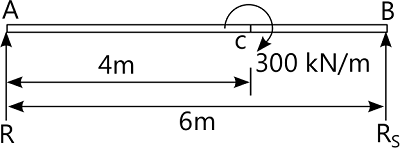16 M

8 (a) Derive torsion equation with usual notation. State the assumptions in the theory of pure torsion.
10 M
8 (b) Derive an expression for Euler's buckling load in a column when both ends are fixed.
10 M

More question papers from Mechanics of Materials